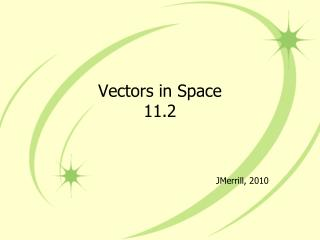DownloadDownload PresentationVectors in Space 11.2

# Vectors in Space 11.2

Télécharger la présentation## Vectors in Space 11.2

- - - - - - - - - - - - - - - - - - - - - - - - - - - E N D - - - - - - - - - - - - - - - - - - - - - - - - - - -
##### Presentation Transcript

1. Vectors in Space11.2 JMerrill, 2010

2. Rules • The same rules apply in 3-D space: • The component form is found by subtracting the coordinates of the initial point from the corresponding coordinates of the terminal point. • Two vectors are = iff their corresponding components are =. • The magnitude (length) of v • Vector addition still means you add the value in the xplace, the y place, and the z place

3. Rules • Scalar multiplication still means you distribute the scalar over the component form • The dot product of • If the dot product = 0, then the vectors are orthogonal. • The angle between two nonzero vectors u and v is

4. Finding the Component Form of a Vector • Find the component form and magnitude of vector v having an initial point of (3,4,2) and a terminal point of (3,6,4) • The component form is • The magnitude is

5. Finding the Dot Product of Two Vectors • Find the dot product of and • Remember the dot product is a scalar, not a vector.

6. Find the Angle Between Two Vectors • Find the angle between u = and v =

7. Parallel Vectors • Vector w has an initial point (1,-2,0) and a terminal point (3,2,1). Which of the following vectors is parallel to w? • A. u = <4,8,2> B. v = <4,8,4> • Put w into component form <2,4,1> • Vector u is the answer because it is just double vector w. U can be written as 2<2,4,1>

8. Using Vectors to Determine Collinear Points • Determine whether the points P(2,-1,4), Q(5,4,6), and R(-4,-11,0) are collinear. • The points P,Q, and R are collinear iff the vectors PQ and PR are parallel. • Because PR = -2PQ, the vectors are parallel. • Therefore, the points are collinear.

9. Finding the Terminal Point of a Vector • The initial point of v = <4,2,-1> is P(3,-1,6). What is the terminal point? • We use the initial point of P(3,-1,6) and the terminal point of Q(q1, q2, q3) • PQ = <q1–3, q2 -(-1), q3–6> = <4,2,-1> • The 1st term = the 1st term, the 2nd term = the 2nd term… So, q1–3 = 4, q2 -(-1) = 2, q3–6 = -1 • Point Q = (7, 1, 5)